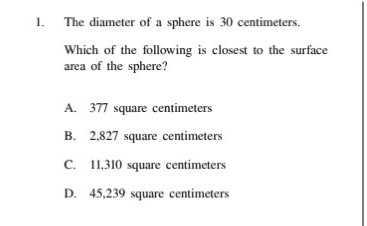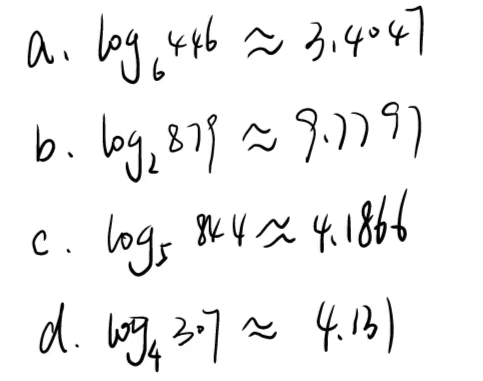### ¿Todavía tienes preguntas de matemáticas?

Pregunte a nuestros tutores expertos
Algebra
Pregunta1. The diameter of a sphere is $$30$$ centimeters. Which of the following is closest to the surface area of the sphere?

A. $$377$$ square centimeters

B. $$2,827$$ square centimeters

C. $$11,310$$ square centimeters

D. $$45,239$$ square centimeters